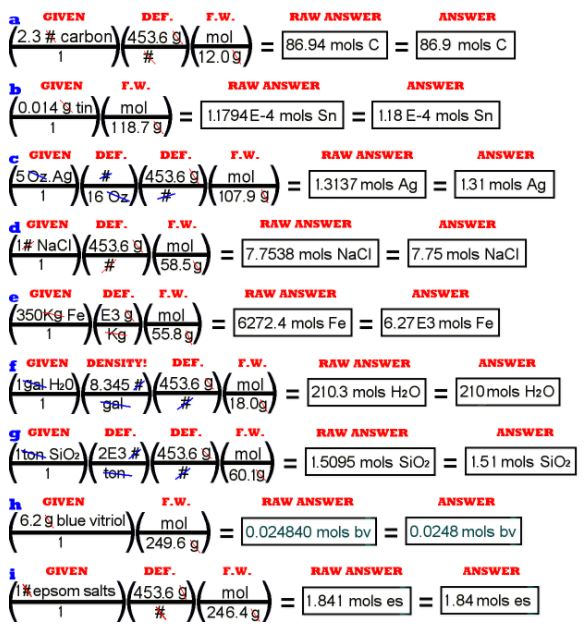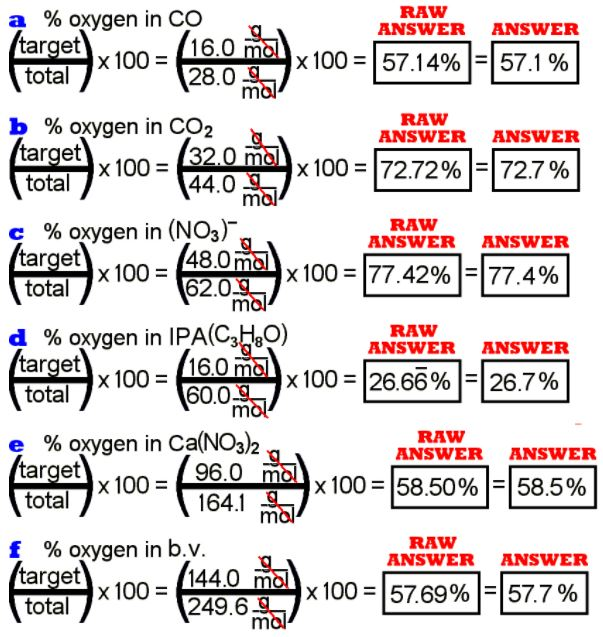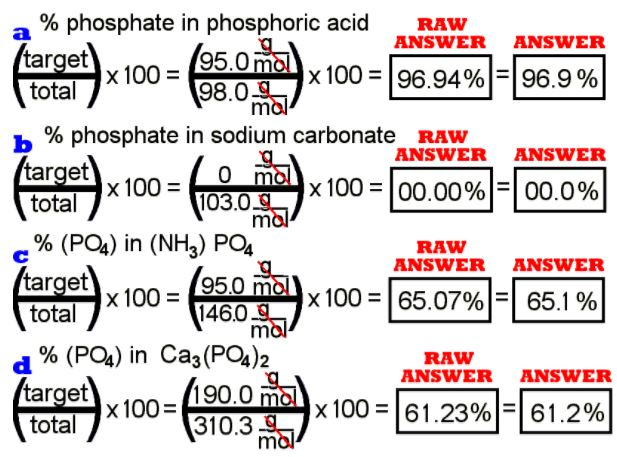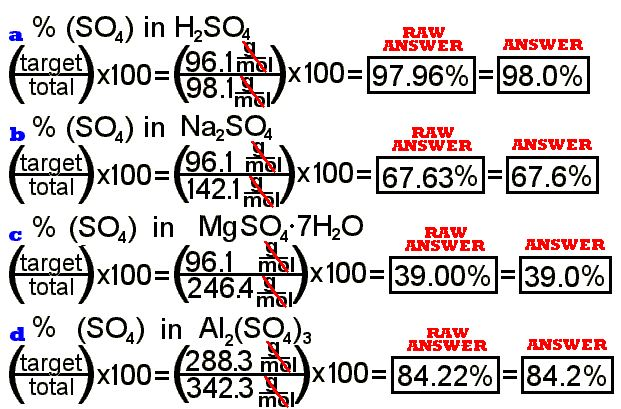PROBLEM #1, SET 1 – MOLS

1. How many pennies are in a mole of pennies? How many thousand-dollar
bills (k-notes!) is that mole of pennies equal to in money value?

The first part of the problem is easy based on the definiton that a mol of
ANYTHING is 6.022 E23 of them. A mol of pennies is 6.022 E23 pennies. This is
what is GIVEN in the second part of the question. The second part is just a
DA problem because we are going from one amount
of money to another.

Pennies (E-2 dollars) to k-notes (E3 dollars) is a metric conversion. Just
from the definitions of penny and k-note, we can say that 1 K-note = E5 pennies.

As for any DA problem, begin with what you know.
There is no denominator for “GIVEN,” so use “1.” Use the definition as a conversion
factor so you can cancel the units you don’t want and leave the units you do.E5 is 100,000. The calculator math is: 6.022 E23 ÷ 100000 =
OR               6.022 E23 ÷ 1 E5 =

The math in this coversion is not difficult, but it is only a reminder of how to do DA problems, the answer is important for its magnitude. Consider
that if we had a mol of pennies, the value of them would be six billion (E9)
times a billion (E9) thousand dollar bills(E5 pennies). This gives you some
idea that the number for mol is so large that it is incomprehensible to most
people. The ease of writing Avogadro’s number in the scientific notation
system gives us a sense of understanding that number that is really not there.

PROBLEM #2, SET 1 – MOLS

2. NO2 is the molecular formula for nitrous dioxide (also
known as nitrogen dioxide). List the information available to you from this formula.

The small formula NO2 has a lot of information in it.
The most basic is that: (a) each formula of nitrous dioxide has one nitrogen
atom and two oxygen atoms, (b) the formula weight is 46 g/mol (16 g/mol for
each oxygen and 14 g/mol for the single nitrogen in each formula), and (c) the
material makes molecules in which the atoms are attached by covalent bonds
(because it is two non – metal elements).

(In answer to part a) Each element has one and only one capital letter. Nobelium has the symbol, “No.”
One capital letter, one element. Carbon monoxide, a compound of one carbon atom
and one oxygen atom, is CO, but cobalt, the single atom of an element, is Co.
In CO2, the smaller subscript “2” after the “O” for oxygen indicates that there are two
oxygen atoms in the formula. The numbers written in this way refer ONLY to the
element just before it OR the group of elements inside the parentheses just before it, such as:
Al2(SO4)3.
In the aluminum sulfate formula the “2” shows two aluminum atoms, the “4” shows
four oxygen atoms, and the “3” outside the parehtheses indicates three phosphates,
that is, the entire contents of the parentheses must be multiplied by three.
The total atom count for aluminum phosphate is two aluminum atoms, three sulfur
atoms, and twelve (three times four) oxygen atoms.

(In answer to part b) One of the important lessons of this chapter is the finding of a formula
weight. The formula weight is the mass in Atomic Mass Units of one entire formula
or the number of grams of material based on the sum of the atomic weights of
the atoms in a formula. (The phrase, “formula weight” is the more general term
that inculdes the term, “molecular weight.” Some materials just don’t come in
molecules.) The formula weight of a material can be found by adding the atomic
weights of the atoms in the a formula of the material. In the case of NO2,
there is one nitrogen at an atomic weight of 14.01 and two oxygen atoms at
atomic weights of 16.00 each. When the formulas become larger, such as the Cu(SO4) · 5H2O, blue
vitriol, in problem #4 m in this set, it might be a good idea to make a list of
the atoms in the material such as:

1 Cu —- 1 x 63.55 = 63.55 g/mol
1 S —– 1 x 32.07 = 32.07 g/mol
4 O —– 4 x 16.00 = 64.00 g/mol
10 H —- 10 x 1.01 = 10.10 g/mol
5 O —– 5 x 16.00 = 80.00 g/mol
__________________________________
totals of 21 atoms and 249.72 g/mol in the formula.

(In answer to part c) A metal and a non-metal will usually form an ionic
bond. An electron or several electrons from the metal leave the metal, forming
a positive ion, and an electron or several electrons can be taken up by the
non – metal to form a negativ ion. The resulting (+ and -) ions attract each
other in what is called an ionic bond. But NO2 has no metal
element in it. The two non – metals can only bond by sharing electrons in a
covalent bond.

PROBLEM #3, SET 1 – MOLS

3. C2H2 is the molecular formula
for ethylyne (A.K.A. acetylene). (a) How many atoms are in one molecule?
(b) Which atoms make up acetylene? (c) How many moles of atoms are in one
molecule of acetylene? (d) How many molecules are in 5.3 moles of acetylene?
(e) How many atoms are in a mole of acetylene?

(a) Acetylene has four atoms in each formula, two carbons and two hydrogens.
It is a true molecule because it is held together by covalent bonds.

(b) Carbon and hydrogen only.

(c) There are four atoms in each molecule of acetylene, so there are 4/Avogadro’s
number of mols of atoms per molecule.
The math is:   4 ÷ 6.022 E 23 = 6.642311524 E-24
mols of atoms per molecule. Round that to 6.6423 E-24 mols of atoms per molecule.

(d) Each mol of acetylene is 6.022 E23 molecules, so 5.3 mols is 5.3 X 6.022 E23
molecules of acetylene.
The math is:   5.3 x 6.022 E 23 = 3.19166 E 24 molecules. Round that to 3.2 E 24 molecules.

(e) Each mol of acetylene is 6.022 E23 molecules, and each molecule has four
atoms, so there are 4 x 6.022 E23 atoms in a mol.
The math is:   4 x 6.022 E 23 = 2.4088 E 24 atoms per mol of acetylene. (Note the number 4 is an exact number, so the answer does not need rounding.)

PROBLEM #4, SET 1 – MOLS

4. Calculate the molar mass of a mole of the following
materials: (a) Al (b) Ra (c) Co (d) CO (e) CO2 (f) HCl (g) Na2CO3
(h) Ca(NO3)2
(i) (NH4)3(PO4)

(j) H2O (k) Epsom salts –
Mg(SO4)Ã‚Â·7H2O (m)
blue vitriol – Cu(SO4) · 5H2O

All of these molar masses can be calculated with
the help of a periodic chart with the atomic weight. The formula weight is the
sum of the atomic weights of the atoms in a formula of the material. The units
of formula weight or atomic weight or molecular weight is “grams per mol.” Chemtutor
calculates the formula weights by using the atomic weight of elements with ONE
DIGIT to the right of the decimal point. Your instructor may use two or three
digits to the right of the decimal point or some other method. It is common to
calculate to the nearest whole number, but many elements have fractional numbers
a significant portion of a whole number. In calculating one decimal to the
right, the most significant deviation is that of hydrogen in organic compounds,
not so much due to the significance of deviation of one atom, but that there
are usually so many hydrogen atoms in oranic compounds.

(a) Al — 1 x 27.0 = 27.0 g/mol

(b) Ra — 1 x 226.0 = 226.0 g/mol

(c) Co — 1 x 58.9 = 58.9 g/mol

(d) CO — C — 1 x 12.0 = 12.0 g/mol
O — 1 x 16.0 = 16.0 g/mol
———————————————–
totals —– 1 C and 1 O —- 28.0 g/mol

(e) CO2 –C — 1 x 12.0 = 12.0 g/mol
O — 2 x 16.0 = 32.0 g/mol
———————————————–
totals —- 1 C and 2 O —- 44.0 g/mol

(f) NaCl — Na — 1 x 23.0 = 23.0 g/mol
Cl — 1 x 35.5 = 35.5 g/mol
———————————————–
totals —- 1 Na and 1 Cl —- 58.5 g/mol

(g) Na2CO3 — Na — 2 x 23.0 = 46.0 g/mol
C — 1 x 12.0 = 12.0 g/mol
O — 3 x 16.0 = 48.0 g/mol
———————————————–
totals —– 2 Na, 1 C, 3 O —– 106.0 g/mol

(h) Ca(NO3)2 — Ca — 1 x 40.1 = 40.1 g/mol
N — 2 x 14.0 = 28.0 g/mol
O — 6 x 16.0 = 96.0 g/mol
———————————————–
totals —— 1 Ca, 2 N, 6 O —— 164.1 g/mol

(i) (NH4)3(PO4) — N — 3 x 14.0 = 42.0 g/mol
H —– 12 x 1.0 = 12.0 g/mol
P —– 1 x 31.0 = 31.0 g/mol
H —– 4 x 16.0 = 64.0 g/mol
———————————————–
totals ——— 1 N and 3 H ——– 149.0 g/mol

(J) H2O — H — 2 x   1.0 =   2.0 g/mol
O — 1 x 16.0 = 16.0 g/mol
———————————————–
totals —- 2 H and 1 O —- 18.0 g/mol

(k) Epsom salts, Mg(SO4)Ã‚Â·7H2O
images/xmol1_1.gif Mg —   1 x 24.3 =   24.3 g/mol
S —–   1 x 32.1 =   32.1 g/mol
H —– 14 x  1.0 =   14.0 g/mol
O —– 11 x 16.0 = 176.0 g/mol
———————————————–
totals —- 1 Mg, 1 S, 14 H, 11 O — 246.4 g/mol

(k) blue vitriol – Cu(SO4) · 5H2O
Cu —   1 x 63.5 =   63.5 g/mol
S —–   1 x 32.1 =   32.1 g/mol
H —– 10 x  1.0 =   10.0 g/mol
O —–  9 x 16.0 = 144.0 g/mol
———————————————–
totals —– 1 Cu, 1 S, 10 H, 9 O —- 249.6 g/mol

PROBLEM #5, SET 1 – MOLS

5. Calculate the number of moles in: (a) 2.3 # of carbon (b)
0.014 g of Tin (c) a 5 Oz silver bracelet (d) a pound of table
salt (e) a 350 Kg cast iron engine block (f) a gal. of water (8.3
#) (g) a ton of sand (SiO2) (h) 6.2 grams of
blue vitriol (i) a pound of Epsom salts

Here for the first time we will be using
DA method to change between mass and mols of a
material. The conversion factor will be the formula weight of the material.
There may be a little more DA to get the amount of material into units of
grams, but that is just added to the main idea, that being that the formula
weight is the way to get from mass to mols or from mols to mass of any
material. Remember that the units of formula weight are grams per mol. Use the

Another way of thinking of the using the formula weight for a conversion
factor is that for every material, the formula weight of it in grams is equal
to a mol of that material.

The only real differences among these problems are the different formula
weights of the materials and different ways to express the given amount of
the material in grams so that they can be converted to mols.Problem 5 f has an interesting part to it. The amount of material was given
in volume rather than mass. An English volume, at that. The way shown here is via
the English weight density of the water. There are several ways to do this, but
I knew the number to make it quicker. The metric density of water is 1 g/cc, but
getting the volume from gallons to cc’s can be a pain. Try it yourself.

PROBLEM #6, SET 1 – MOLS

6. Calculate the number of atoms in: (a) 100 g of Argon (b)
1.21 Kg aluminum foil (c) a 28 # lead brick (d) the E7 Kg of water in
an olympic swimming pool (e) 7 Kg of hydrogen gas (f) a tonne of
calcium nitrate

Now we can investigate the relationship between mols
and number of molecules or atoms. You could call the units of Avogadro’s Number
atoms per mol or molecules per mol or just formulas per mol, depending upon
how the material in question naturally comes. A mol of the element tin is
Avogadro’s number of atoms because elements come in atoms. A mol of water is
Avogardo’s number of molecules because water doesn’t come any other way. A mol
of blue vitriol, Cu(SO4)Ã‚Â·5H2O,
is Avogogadro’s number of the whole formula, to include the water parts. Even though
the formula comes apart into ions in solution and the water parts are not
chemically attached, the entire group of atoms makes up a formula.”You can see that we have to calculate the number of UNITS of the material,
whatever units it naturally comes in, and then consider the number of atoms
in each unit of the material. (I have not given the raw answers in some of the
problems above to save space.)

PROBLEM #7, SET 1 – MOLS

7. What is the percentage composition of oxygen in each of the
following materials: (a) CO (b) CO2 (c)
(NO3)
(d) isopropyl alcohol C3H8O
(e) calcium nitrate (f) blue vitriol Cu(SO4)Ã‚Â·5H2O

Percentage is defined as: the target over the total times 100.
Percentage composition is a common statistical treatment for a subset of a
material within a larger material, for instance the percentage of sand in a
concrete mixture or the percentage of males under twelve years old in the
population of the United States populaion.PROBLEM #8, SET 1 – MOLS

8. What is the percentage composition of phosphate in each of
the following materials: (a) phosphoric acid (b) sodium
carbonate (c) ammonium phosphate (d) aluminum phosphate